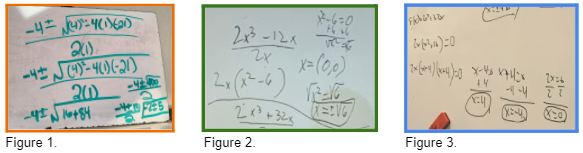# IMPACT Live!

View Only

### IMPACT Plus - Analyzing Student Work

#### By April Strom posted 01-18-2022 08:49:14

As we imagine the learning of mathematics as an active and engaging experience for students, we need to leverage what we know from research which encourages the use of student thinking to guide instruction. Instructional strategies, such as the use of “vertical non-permanent surfaces” (VNPS) as promoted in Building Thinking Classrooms by Peter Liljedahl, provide an essential window into student thinking. So, what does it mean to use student thinking when teaching? And in what ways can student thinking guide instruction?

Let’s explore a traditional task first and then discuss thoughts on these questions. Consider the following task: Solve the following using the quadratic formula: x2+4x-21=0.Imagine a classroom where students are working in pairs to construct the solutions to the above quadratic equation. Students show work on their whiteboards and one pair displays the work shown in Figure 1. What thinking is displayed in this image? More importantly, what thinking is not displayed in this image that you, as the instructor, and students in the classroom would be interested in knowing? Now fast forward to another class session where the following task is posed: Find the zeros of f(x)=2x3-12x. After several minutes, a small group of students show their solution on a whiteboard (see Figure 2). What advice would you give to the student (or class) about the work presented in Figure 2? What thinking is not displayed here that you would like to highlight for the students who produced this work? Finally, imagine that students completed a similar problem, but this time they are asked to find the zeros of f(x)=2x3+32x. A group of students produced the work shown in Figure 3. What teacher moves would you make in the moment of teaching that leverages student thinking based on the work shown?As we teach, we encounter critical moments where we analyze student work, diagnose their thinking, and then determine the teacher moves to elevate productive and non-productive ways of thinking for the purpose of deepening understanding. As you review the samples of student work in Figures 1-3, consider what you would choose to highlight for the class.

Using vertical non-permanent surfaces to highlight student thinking is a powerful tool for making student thinking public so that you, as the instructor, can pinpoint the conceptual victories that students are able to showcase. But, more importantly, it allows you to diagnose mathematical errors and imprecisions. In the case of Figure 1, the student was able to obtain the correct answers, albeit not simplified. However, the imprecise mathematical notation with the absence of both x and the equal signs calls into question whether the student understands the meaning of the solutions they found. Similarly, in the case of Figure 2, while the work shown indicates that the students seem to understand the process of solving the given cubic equation, they did not attend to mathematical precision in setting up an initial equation that would prompt one to continue solving. Yet, the students were able to appropriately and precisely set up the equation x2-6=0 and generate the solutions x=6. However, the students then wrote another solution as x=(0,0), which is notationally incorrect. Finally, in the case of Figure 3, the students ignored that they needed to factor x2+16 and instead they factored x2-16 and moved forward in solving the equation. Clearly, the solutions in Figure 3 are incorrect but the students were unaware of their error, especially since they did not check their solutions algebraically or graphically.

The examples of student work displayed in Figures 1-3 highlight common mathematical errors and imprecisions. The question we want to consider, though, is how do we as instructors use this evidence of student thinking to move students’ mathematical conceptions and abilities forward? Do we take the time in class to pause and call attention to mathematical errors and imprecisions in a way that promotes learning? Do we allow students the opportunity to critique the mathematical reasoning of other students? Do we leverage students’ mathematics as a conceptual springboard to further develop students’ understanding of mathematics? These are questions that need our attention and reflection as we grapple with instructional practices that truly support learning.

Teaching mathematics begins once students’ thinking is made public both verbally and in writing and continues as we leverage the thinking to further mathematical ideas. In the examples provided in Figures 1-3, the instructor I was observing did not leverage students’ mathematical work to make in-the-moment affirmations about the mathematics displayed as a way to further understanding. Rather, the instructor simply moved on to other examples. It was as if the goal was merely to have students put their thinking on the whiteboard without allowing time or space for critiquing the thinking by either the instructor or other students in the class. This puzzled me and prompted me to reflect on the ways in which we can probe student thinking by leveraging their own work when showcased publicly. This is the ultimate goal of teaching — and for learning — mathematics! As we teach for understanding through active learning, we can engage the students in critiquing the mathematical reasoning of others by asking these starter questions:

1. What do you appreciate about the work shown?
2. Are you convinced by the mathematics shown that the solution is correct?
3. What advice would you give to improve the work shown?

The goal is for students to share what they value and appreciate about the work shown by other students, then move to providing a critique of the solution that can improve the potential of the solution to be a correct and convincing mathematical argument. Through attending to precision and leveraging students’ thinking as the springboard for teaching and learning, students will have opportunities to engage in active learning, active listening, and meaning-making of mathematics. This is when the magical and beautiful nature of mathematics comes to life!